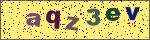常用js正则表达式

http://www.w3schools.com/js/js_obj_regexp.asp

/^\s*\$/

/\d{2}-\d{5}/

/<\s*(\S+)(\s[^>]*)?>[\s\S]*<\s*\/\1\s*>/

\

^

\$

*

+

?

{n}

n 是非负整数。正好匹配 n 次。例如，“o{2}”与“Bob”中的“o”不匹配，但与“food”中的两个“o”匹配。

{n,}

n 是非负整数。至少匹配 n 次。例如，“o{2,}”不匹配“Bob”中的“o”，而匹配“foooood”中的所有 o。“o{1,}”等效于“o+”。“o{0,}”等效于“o*”。

{n,m}

M 和 n 是非负整数，其中 n <= m。匹配至少 n 次，至多 m 次。例如，“o{1,3}”匹配“fooooood”中的头三个 o。'o{0,1}' 等效于 'o?'。注意：您不能将空格插入逗号和数字之间。

?

.

(pattern)

(?:pattern)

(?=pattern)

(?!pattern)

x|y

[xyz]

[^xyz]

[a-z]

[^a-z]

\b

\B

\cx

\d

\D

\f

\n

\r

\s

\S

\t

\v

\w

\W

\xn

\num

\n

\nm

\nml

\un

"^/d+\$"　　//非负整数（正整数 + 0）
"^[0-9]*[1-9][0-9]*\$"　　//正整数
"^((-/d+)|(0+))\$"　　//非正整数（负整数 + 0）
"^-[0-9]*[1-9][0-9]*\$"　　//负整数
"^-?/d+\$"　　　　//整数
"^/d+(/./d+)?\$"　　//非负浮点数（正浮点数 + 0）
"^(([0-9]+/.[0-9]*[1-9][0-9]*)|([0-9]*[1-9][0-9]*/.[0-9]+)|([0-9]*[1-9][0-9]*))\$"　　//正浮点数
"^((-/d+(/./d+)?)|(0+(/.0+)?))\$"　　//非正浮点数（负浮点数 + 0）
"^(-(([0-9]+/.[0-9]*[1-9][0-9]*)|([0-9]*[1-9][0-9]*/.[0-9]+)|([0-9]*[1-9][0-9]*)))\$"　　//负浮点数
"^(-?/d+)(/./d+)?\$"　　//浮点数
"^[A-Za-z]+\$"　　//由26个英文字母组成的字符串
"^[A-Z]+\$"　　//由26个英文字母的大写组成的字符串
"^[a-z]+\$"　　//由26个英文字母的小写组成的字符串
"^[A-Za-z0-9]+\$"　　//由数字和26个英文字母组成的字符串
"^/w+\$"　　//由数字、26个英文字母或者下划线组成的字符串
"^[/w-]+(/.[/w-]+)*@[/w-]+(/.[/w-]+)+\$"　　　　//email地址
"^[a-zA-z]+://(/w+(-/w+)*)(/.(/w+(-/w+)*))*(/?/S*)?\$"　　//url
/^(d{2}|d{4})-((0([1-9]{1}))|(1[1|2]))-(([0-2]([1-9]{1}))|(3[0|1]))\$/ // 年-月-日
/^((0([1-9]{1}))|(1[1|2]))/(([0-2]([1-9]{1}))|(3[0|1]))/(d{2}|d{4})\$/ // 月/日/年
"^([w-.]+)@(([[0-9]{1,3}.[0-9]{1,3}.[0-9]{1,3}.)|(([w-]+.)+))([a-zA-Z]{2,4}|[0-9]{1,3})(]?)\$" //Emil
"(d+-)?(d{4}-?d{7}|d{3}-?d{8}|^d{7,8})(-d+)?" //电话号码
"^(d{1,2}|1dd|2[0-4]d|25[0-5]).(d{1,2}|1dd|2[0-4]d|25[0-5]).(d{1,2}|1dd|2[0-4]d|25[0-5]).(d{1,2}|1dd|2[0-4]d|25[0-5])\$" //IP地址

发表评论